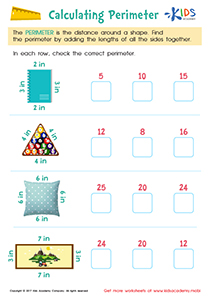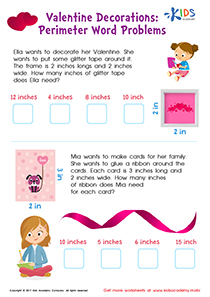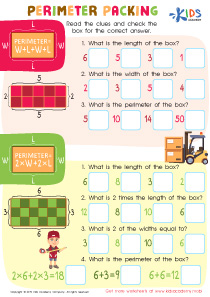# Math Lesson - Understanding Perimeter, Grade 3

• ### Activity 1 / Calculating Perimeter WorksheetSneak a peek at this calculating perimeter worksheet for grade 3, and see how kids learn this useful geometric skill!
This worksheet will help kids find the perimeter of everyday objects pictured on the sheet. By practicing, kids build important math skills they’ll use to master complex geometry!

• ### Activity 2 / Perimeter Word Problems WorksheetLearners practice perimeter in a fun and realistic way while celebrating Valentine’s Day!
This perimeter word problems worksheet for 3rd grade offers valuable practice with tricky word problems as children solve problems to determine how much material is needed to make pretty valentines.

• ### Activity 5 / Perimeter Parking WorksheetGeometry is one aspect of mathematics that your kids might not be too excited about, but it is up to you to show them how easy geometry problems can really be. With simple exercises and familiar objects, your kids can become better with solving geometry problems. In this worksheet there are two sections each with different questions. Help your kids read the clues and then check the box which has the correct answer.

?